# Linux kernel hugepage allocation latencies

## A detailed analysis

Some drivers needs to allocate almost all memory as hugepages to reduce (on-device or CPU) TLB pressure. However, on a running system, higher order allocations can fail if the memory is fragmented. Linux kernel can do on-demand compaction as we request more hugepages but this style of compaction incurs very high latency.

To show the effect of on-demand compaction on hugepage allocation latency, I created a test program “frag” which allocates almost all available system memory followed by freeing $\frac{3}{4}$ of pages from each hugepage-sized aligned chunk. This allocation pattern results in ~300% fragmented address space w.r.t order 9 i.e. physical mappings of our VA space is spread over 3x the number of hugepage-aligned chunks than what is ideally required.

In actual numbers, on a system with 32G RAM, frag allocates a total of 28G of RAM (somewhat short of 32G, to avoid running into the OOM killer) and after unmapping pages in the above manner, it ends up with 7G of memory ($\frac{3}{4} \times 32G$) allocated. At this stage, this 7G of memory is spread across (almost) entire 32G physical memory, leaving no hugepage aligned chunks available for allocation (i.e. PA space fragmentation w.r.t order-9 is 100% at this stage).

With the system memory in such a fragmented state, a test kernel driver “khugehog” then tries to allocate as many 2M hugepages as possible (with GFP_KERNEL allocation flag) and records latency for each allocation.

It is interesting to visualize the physical address space at difference stages of our experiment – here is how the physical memory layout looks like just after running the frag program. I got this layout map by walking frag’s page tables, dumping VA->PA mappings, sorting PAs and then binning them in 2M aligned regions: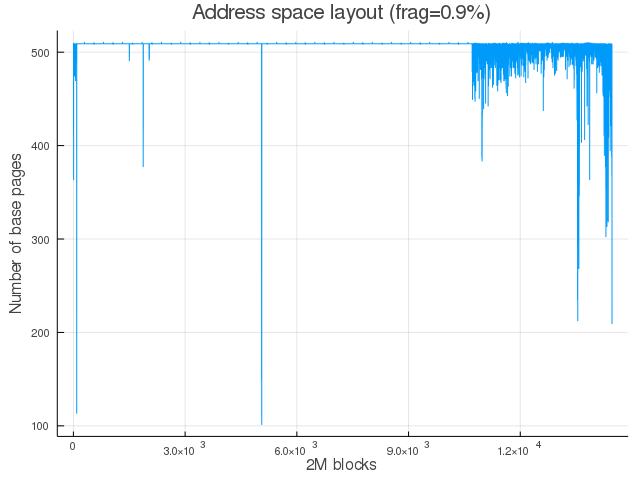This figure shows number of 4K pages within each 2M ( = 512 x 4K pages) aligned physical region that are mapped to the frag process. Since frag is the only major program running on the test system, this plot gives fairly accurate view of the entire PA space mapping. The plot shows ~512 pages mapped for each 2M region (except for some noise in 2M blocks near the end). This is the ideal state of mapping since we are using each 2M chunk optimally by mapping all its 512 x 4K component pages.

In the second stage, we unmap $\frac{3}{4}$ of pages from each 2M aligned chunk (as I have described above) which results in physical address space layout like this: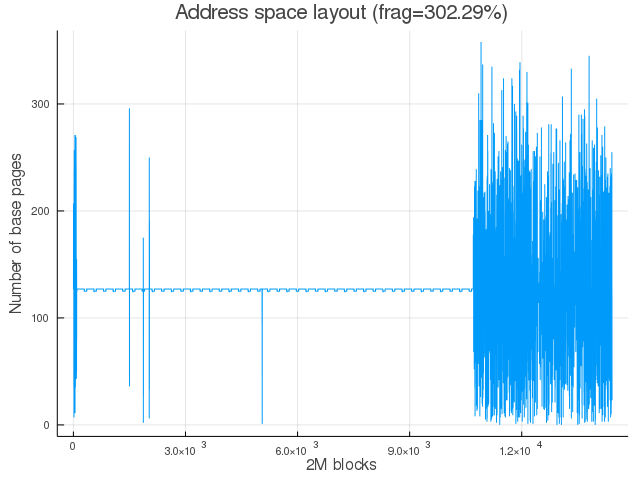Now, each 2M block has only ~128 x 4K pages mapped (except for some noise for 2M blocks at the end). This plot shows that, at this stage, almost no 2M hugepage block is fully available for allocation. This test program simulates a typical long running server where physical space gets randomly mapped with lesser number of physically contiguous blocks (especially for orders > 9) available over time.

If a subsystem/driver requests hugepages with memory in such a state, the Linux kernel uses a technique called compaction which remaps physical pages such that larger physical blocks are eventually available for allocation. You should definitely read this LWN.net article on compaction which gives a great explanation of this concept.

This compaction process can very expensive. It has to unmap pages, issue page-level TLB flushes, copy pages to another location, establish new page-table mappings. To reduce the performance impact, the Linux kernel does compaction only when really needed: typically when a hugepage allocation would fail but there are enough total free pages available – in such cases, it compacts the memory to make one higher-order (order of the failing allocation) worth of contiguous physical block available, to make that allocation succeed.

This kind of on-demand compaction scheme seems really effective (as we will see below) in making hugepages available when needed but at the cost of very high latencies. The following plot shows allocation latencies as reported from the test kernel module (khugehog) after the userspace test program (frag) has fragmented the physical address space (see Fig 2).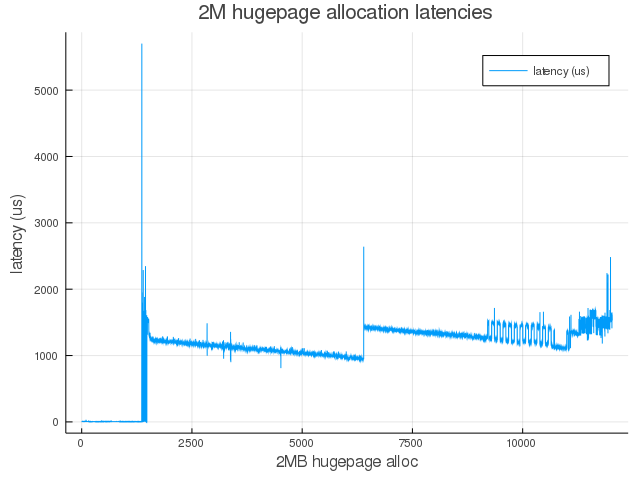Here is the summary of data shown in the above plot:

StatLatency ($\mu$s)
count$12032$
min$2.0$
max$5701.0$
$\mu$$1090.61 \sigma$$426.05$

The same data as percentiles:

percentilelatency ($\mu$s)
$5$$5 10$$5$
$25$$1047 30$$1080$
$40$$1131 50$$1189$
$60$$1243 75$$1346$
$80$$1372 90$$1436$
951484 • Userspace frag has 7G of memory allocated at this stage (\frac{1}{4} \times 28G). So, total available memory is 32G - 7G = 25G. • Kernel module khugehog could allocate 12032 \times 2M = 23.5G which is 94% of current free memory. Above data shows that on-demand compaction was really effective in making hugepages available for allocation but at the cost of very high latencies. It’s interesting to visualize the physical address space layout after the khugehog stage: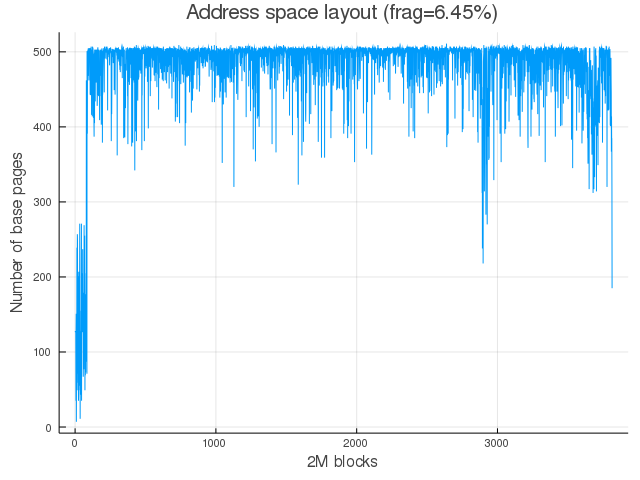The plot show ~500 pages mapped within each 2M block. This is very close to the ideal mapping of 512 pages per 2M block. Compare this with Fig 1 which shows physical address space layout before the program starts punching holes to fragment the space. Comparing the two images, we can see that with on-demand compaction we did somewhat converge to the ideal mapping state. Above latency numbers would make more sense if we have a baseline to compare against. Let’s see how these latency numbers change if we trigger a manual memory compaction just before the khugehog step. In summary this is what we will do: 1. Run frag to allocate 28G (or 32G total) RAM 2. Unmap \frac{3}{4} of pages from each 2M aligned region 3. Manually compact memory with: echo 1 | sudo tee /proc/sys/vm/compact_memory. This whole memory compaction took <1 sec. 4. Trigger khugehog to allocate as many hugepages as possible and report allocation latencies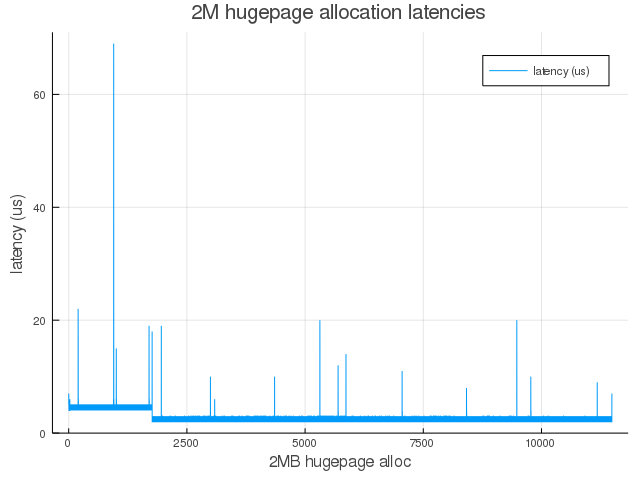Again, let’s summarize data shown in this plot: StatLatency count11489 min2.0 max69.0 \mu2.7
$\sigma$$1.27 The same data as percentiles: percentilelatency 5$$2$
$10$$2 25$$2$
$30$$2 40$$2$
$50$$2 60$$3$
$75$$3 80$$3$
$90$$5 95$$5$

The above data excludes a single allocation with latency 12517 $\mu$s which was observed just before khugehog observed allocation failure (which stop the alloc/measure loop).

Comparing data for on-demand compaction vs data after one-time manual compaction, we see, for example, 90th percentile latency drop from 1426 $\mu$s to just 5 $\mu$s – a difference of several orders of magnitude.

Note: The workload we used in our experiments to trigger fragmentation (the frag program) creates a memory state which is very easy to compact. In a more realistic scenario, the physical memory may be scattered with “non-movable” pages which cannot be moved around during compaction. This kind of workload is intentional as it gives us a lower bound on compaction overhead as measured in terms of hugepage allocation latency.

# Summary

Linux kernel does on-demand memory compaction in response to failing higher-order allocations. This scheme is often effective in making the allocation succeed but incurs a very high average latency. Experiment with manually triggering compaction shows dramatically lower latencies as we no longer hit compaction on allocation path. Also noteworthy is the fact that manual compaction took <1 sec on a 32G system.

Linux kernel community is aware of these issues and various developers have suggested Proactive compaction as a possible solution. Here is my implementation for the same as a kernel patch. I hope to cover proactive compaction in detail in a future post.